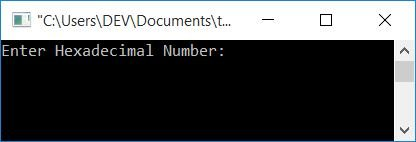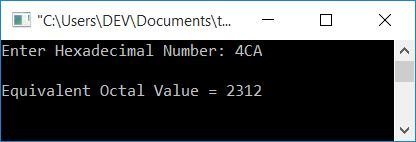# C Program to Convert Hexadecimal to Octal

In this article, you will learn and get code about the conversion of a number given by the user (at run-time) from hexadecimal to octal.

Before going to the program, if you are not aware of:

• Octal Number

Then refer to the Hexadecimal to Octal conversion step-by-step process. Now let's move on to the program.

## Hexadecimal to Octal in C

To convert a hexadecimal number to an octal number in C programming, you have to ask the user to enter the hexadecimal number and then convert it into its equivalent octal value. And then display the equivalent value in octal, as shown in the program given below.

Note: There is no direct conversion from hexadecimal to octal. Therefore you can prefer either hexadecimal to decimal and then decimal to octal, or hexadecimal to binary and then binary to octal.

Let's create a program that converts hexadecimal to binary and then binary to octal. Later on, the same program is also created in a second way. In my opinion, the second way is easier to create or understand.

```#include<stdio.h>
#include<conio.h>
#include<string.h>
int main()
{
int i=0, chk=0, len, rem, binDigit, octalDigit;
long long binaryInt=0, temp=1, octalNum, binaryNum;
char hexdec, binnum="";
gets(hexdec);
while(hexdec[i])
{
switch(hexdec[i])
{
case '0':
strcat(binnum, "0000");
break;
case '1':
strcat(binnum, "0001");
break;
case '2':
strcat(binnum, "0010");
break;
case '3':
strcat(binnum, "0011");
break;
case '4':
strcat(binnum, "0100");
break;
case '5':
strcat(binnum, "0101");
break;
case '6':
strcat(binnum, "0110");
break;
case '7':
strcat(binnum, "0111");
break;
case '8':
strcat(binnum, "1000");
break;
case '9':
strcat(binnum, "1001");
break;
case 'A':
strcat(binnum, "1010");
break;
case 'a':
strcat(binnum, "1010");
break;
case 'B':
strcat(binnum, "1011");
break;
case 'b':
strcat(binnum, "1011");
break;
case 'C':
strcat(binnum, "1100");
break;
case 'c':
strcat(binnum, "1100");
break;
case 'D':
strcat(binnum, "1101");
break;
case 'd':
strcat(binnum, "1101");
break;
case 'E':
strcat(binnum, "1110");
break;
case 'e':
strcat(binnum, "1110");
break;
case 'F':
strcat(binnum, "1111");
break;
case 'f':
strcat(binnum, "1111");
break;
default:
chk = 1;
break;
}
i++;
}
if(chk==0)
{
len = strlen(binnum);
while(len!=0)
{
if(binnum[len-1]=='0')
binDigit=0;
else
binDigit=1;
binaryInt = binaryInt + (binDigit*temp);
temp = temp*10;
len--;
}
binaryNum = binaryInt;
octalNum = 0;
temp = 1;
while(binaryNum>0)
{
rem = binaryNum%1000;
switch(rem)
{
case 0:
octalDigit = 0;
break;
case 1:
octalDigit = 1;
break;
case 10:
octalDigit = 2;
break;
case 11:
octalDigit = 3;
break;
case 100:
octalDigit = 4;
break;
case 101:
octalDigit = 5;
break;
case 110:
octalDigit = 6;
break;
case 111:
octalDigit = 7;
break;
}
octalNum = (octalDigit*temp) + octalNum;
binaryNum = binaryNum/1000;
temp = temp*10;
}
printf("\nEquivalent Octal Value = %lld", octalNum);
}
else
getch();
return 0;
}```

This program was compiled and executed using the Code::Blocks IDE. Here is the sample run. This is the first snapshot of the sample run:Now supply any input value (as a hexadecimal number), say 4CA, and press the ENTER key to see its equivalent value in the octal number system, as shown in the second snapshot given below:In the above program, we have converted the hexadecimal value to its equivalent binary value. After performing this conversion, we have applied the code given below:

```len = strlen(binnum);
while(len!=0)
{
if(binnum[len-1]=='0')
binDigit=0;
else
binDigit=1;
binaryInt = binaryInt + (binDigit*temp);
temp = temp*10;
len--;
}```

to convert the binary value stored in a character array to an integer. Then I applied the conversion from binary to octal.

### Hexadecimal to Octal in C using Indirect Conversion

This program converts a hexadecimal number (supplied by the user at run-time) to its decimal equivalent. And then the decimal equivalent gets converted into its octal equivalent.

```#include<stdio.h>
#include<conio.h>
#include<math.h>
int main()
{
int decnum=0, rem, i=0, len=0, octnum;
char hexnum;
scanf("%s", hexnum);
while(hexnum[i]!='\0')
{
len++;
i++;
}
len--;
i=0;
while(len>=0)
{
rem = hexnum[len];
if(rem>=48 && rem<=57)
rem = rem-48;
else if(rem>=65 && rem<=70)
rem = rem-55;
else if(rem>=97 && rem<=102)
rem = rem-87;
else
{
printf("\nYou've entered an invalid Hexadecimal digit");
getch();
return 0;
}
decnum = decnum + (rem*pow(16, i));
len--;
i++;
}
i=0;
while(decnum != 0)
{
octnum[i] = decnum%8;
i++;
decnum = decnum/8;
}
printf("\nEquivalent Octal Value = ");
for(i=(i-1); i>=0; i--)
printf("%d", octnum[i]);
getch();
return 0;
}```

For in-depth learning of the above code, you can follow the separate program on hexadecimal to decimal and decimal to octal.

#### The same program in different languages

C Quiz

« Previous Program Next Program »